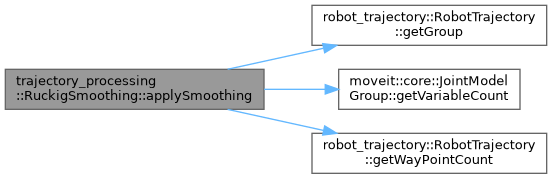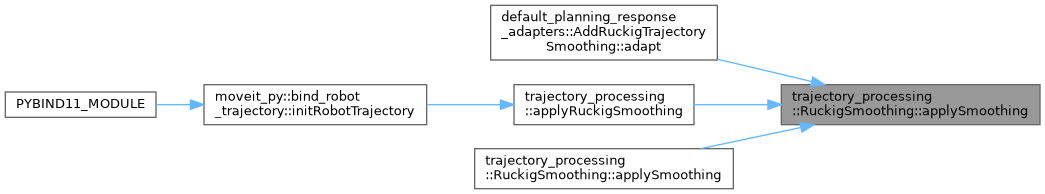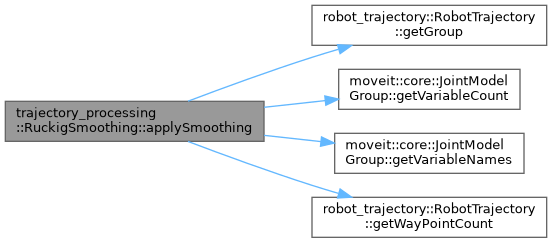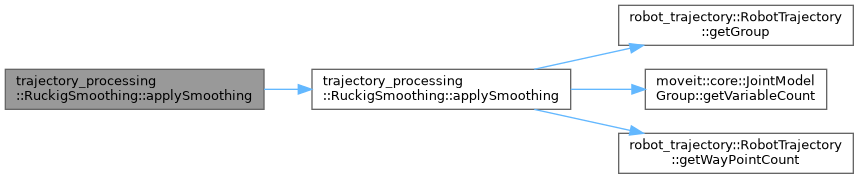moveit2 The MoveIt Motion Planning Framework for ROS 2.
trajectory_processing::RuckigSmoothing Class Reference

`#include <ruckig_traj_smoothing.h>`

## Static Public Member Functions

static bool applySmoothing (robot_trajectory::RobotTrajectory &trajectory, const double max_velocity_scaling_factor=1.0, const double max_acceleration_scaling_factor=1.0, const bool mitigate_overshoot=false, const double overshoot_threshold=0.01)
Apply smoothing to a time-parameterized trajectory so that jerk limits are not violated. More...

static bool applySmoothing (robot_trajectory::RobotTrajectory &trajectory, const std::unordered_map< std::string, double > &velocity_limits, const std::unordered_map< std::string, double > &acceleration_limits, const std::unordered_map< std::string, double > &jerk_limits, const double max_velocity_scaling_factor=1.0, const double max_acceleration_scaling_factor=1.0, const bool mitigate_overshoot=false, const double overshoot_threshold=0.01)
Apply smoothing to a time-parameterized trajectory so that jerk limits are not violated. More...

static bool applySmoothing (robot_trajectory::RobotTrajectory &trajectory, const std::vector< moveit_msgs::msg::JointLimits > &joint_limits, const double max_velocity_scaling_factor=1.0, const double max_acceleration_scaling_factor=1.0)
Apply smoothing to a time-parameterized trajectory so that jerk limits are not violated. More...

## Detailed Description

Definition at line 45 of file ruckig_traj_smoothing.h.

## ◆ applySmoothing() [1/3]

 bool trajectory_processing::RuckigSmoothing::applySmoothing ( robot_trajectory::RobotTrajectory & trajectory, const double max_velocity_scaling_factor = `1.0`, const double max_acceleration_scaling_factor = `1.0`, const bool mitigate_overshoot = `false`, const double overshoot_threshold = `0.01` )
static

Apply smoothing to a time-parameterized trajectory so that jerk limits are not violated.

Parameters
 [in,out] trajectory A path which needs smoothing. max_velocity_scaling_factor A factor in the range [0,1] which can slow down the trajectory. max_acceleration_scaling_factor A factor in the range [0,1] which can slow down the trajectory. mitigate_overshoot If true, overshoot is mitigated by extending trajectory duration. overshoot_threshold If an overshoot is greater than this, duration is extended (radians, for a single joint)
Returns
true if successful.

Definition at line 59 of file ruckig_traj_smoothing.cpp.

Here is the call graph for this function:Here is the caller graph for this function:## ◆ applySmoothing() [2/3]

 bool trajectory_processing::RuckigSmoothing::applySmoothing ( robot_trajectory::RobotTrajectory & trajectory, const std::unordered_map< std::string, double > & velocity_limits, const std::unordered_map< std::string, double > & acceleration_limits, const std::unordered_map< std::string, double > & jerk_limits, const double max_velocity_scaling_factor = `1.0`, const double max_acceleration_scaling_factor = `1.0`, const bool mitigate_overshoot = `false`, const double overshoot_threshold = `0.01` )
static

Apply smoothing to a time-parameterized trajectory so that jerk limits are not violated.

Parameters
 [in,out] trajectory A path which needs smoothing. velocity_limits Joint names and velocity limits in rad/s acceleration_limits Joint names and acceleration limits in rad/s^2 jerk_limits Joint names and jerk limits in rad/s^3 max_velocity_scaling_factor A factor in the range [0,1] which can slow down the trajectory. max_acceleration_scaling_factor A factor in the range [0,1] which can slow down the trajectory. mitigate_overshoot If true, overshoot is mitigated by extending trajectory duration. overshoot_threshold If an overshoot is greater than this, duration is extended (radians, for a single joint)
Returns
true if successful.

Definition at line 90 of file ruckig_traj_smoothing.cpp.

Here is the call graph for this function:## ◆ applySmoothing() [3/3]

 bool trajectory_processing::RuckigSmoothing::applySmoothing ( robot_trajectory::RobotTrajectory & trajectory, const std::vector< moveit_msgs::msg::JointLimits > & joint_limits, const double max_velocity_scaling_factor = `1.0`, const double max_acceleration_scaling_factor = `1.0` )
static

Apply smoothing to a time-parameterized trajectory so that jerk limits are not violated.

Parameters
 [in,out] trajectory A path which needs smoothing. joint_limits Joint names and corresponding velocity limits in rad/s, acceleration limits in rad/s^2, and jerk limits in rad/s^3 max_velocity_scaling_factor A factor in the range [0,1] which can slow down the trajectory. max_acceleration_scaling_factor A factor in the range [0,1] which can slow down the trajectory.

Definition at line 149 of file ruckig_traj_smoothing.cpp.

Here is the call graph for this function:The documentation for this class was generated from the following files: